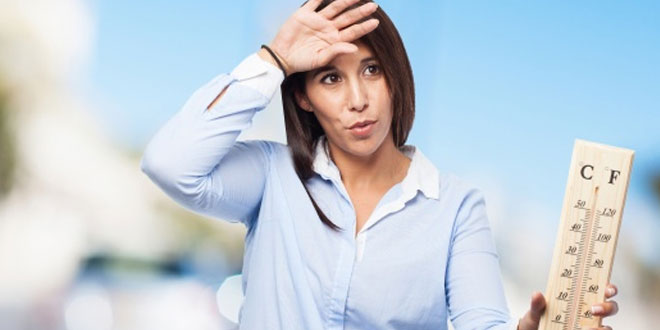Thursday , July 7 2022# NCERT 7th Class (CBSE) Science: Heat and Temperature

#### Question: What are the different thermometer scales?

1. The centigrade or Celsius scale: It is introduced by Anders Celsius, is usually used in scientific laboratories. In this scale the lower fixed point or the ice-point is 0°C and the steam-point or upper fixed point is 100°C. The fundamental interval is divided in 100 equal parts; each part is called 1°C (one degree Celsius).
2. The Fahrenheit scale: It is suggested by Daniel Gabriel Fahrenheit, is usually used in clinical and meteorological purposes. Here, the ice-point and the steam-point are correspondingly marked as 32°F and 212°F, and the fundamental interval is divided into 180 equal divisions. Each division is called one degree Fahrenheit. (1°F).
3. Absolute scale or Kelvin scale: It is designed by Lord Kelvin, is used internationally in modern scientific world. In this scale the ice-point is marked 273K and the steam-point 373K, the fundamental interval is divided into 100 equal divisions, like that in the Celsius scale. Each division is read as one degree absolute or one Kelvin (IK). In fact, Kelvin scale of temperature starts from the temperature corresponding to -273°C, taken as zero Kelvin

#### Question: What is the Relation between Celsius, Fahrenheit and Kelvin scales?

Answer: Since the range of temperature from ice-point to steam-point is equal in all the three scales, 100 centigrade degrees = (212 – 32) or 180 Fahrenheit degrees = (373 – 273) or 100 absolute degrees.

We consider three thermometers in the above three scales are dipped simultaneously in a liquid of certain temperature. Let the temperatures recorded in the Celsius, Fahrenheit and Kelvin thermometers  respectively be C, F and K.

Now it can be proved that  C / 5 = F- 32 / 9 = K- 273 /5

#### Question: What is the unit of heat?

1. C.G.S. unit of heat is Calorie
2. The M.K.S. or S.I. unit of heat is Joule, 1 calorie equals 4.18 or 4.2 joules approximately.

## Sources of Energy: 10th Science Chapter 14

Class: 10th Class Subject: Science Chapter: Chapter 14: Sources of Energy Quiz: – Questions MCQs: …

1.Not so good but it’s OK. Sometimes good for exams.

2.Not bad ha good for me!

3.Nice but not bad useful during exams

•Yes. True, You should add more questions

4.What is the difference between Mercury thermometer and a Digital thermometer ?

5.SO USEFUL FOR MY EXAMS